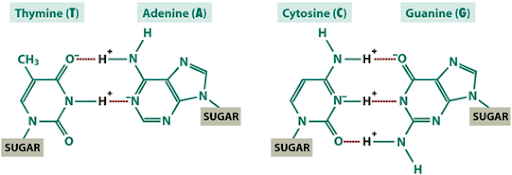# What is Chargaff’s rule or the equivalence rule ?

Chargaff’s rule (the equivalence rule): He found out that in DNA,  the concentration of adenine always equalled the concentration of thymine and the concentration of guanine always equalled the concentration of cytosine ie. the amount of purine=the amount of pyramidine in a given DNA molecule.

(Chargaff's Rule Questions)

In short,
In a given sample of DNA, the total amount of purines = total amount of pyrimidines.
i. e. A+G=T+C
• The ratio of A:T =1
• The ratio of G:C =1
• A/T or G/C=1, A=T, G=C
• A+T=C+G (No. of AT pairs need not equal to the number of GC pairs)• Adenine is paired to Thymine by 2 hydrogen bonds
• Cytosine is paired to Guanine by 3 hydrogen bonds
[A]=[T] and [G]=[C] therefore [A]+[T]not equal to [G]+[C]

A+T/G+C ratio varies with species value <1 GC type as in Mycobacterium 0.6%

>1 AT type as in humans 1.4%

The relative amount of each N2 base present in a DNA sample differ between species to species, but always same within the species (A=T)/(G=C) not equal to one

• In humans 30% A, 30% T, 20% g and 20% C
• In E.coli 24% A, 24% T, 26% G, 26% C